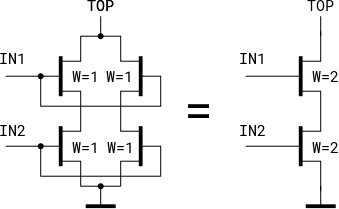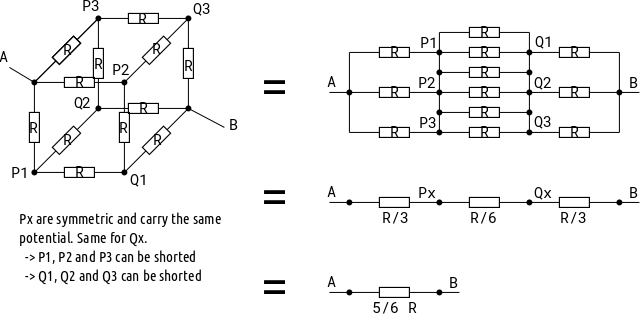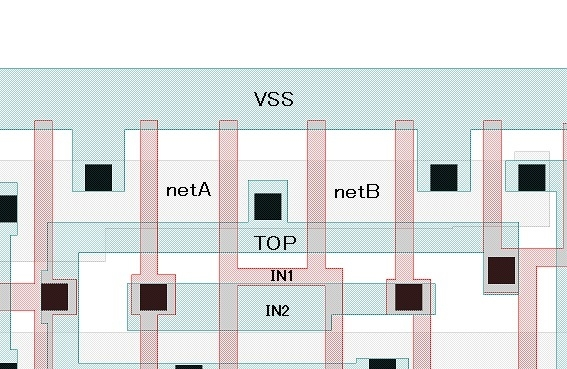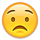# Reduce split gate option in LVS

Hi Matthias,
In LVS using some complex Flip Flop cells, I encountered the case that Klayout required MOS device reduction option for the extracted net list as shown below. (Sorry "Attach image" file failed to upload at present.)
Extracted circuit
M1Up netA IN1 TOP VSS NMOS L=1U W=1U
M1Low netA IN2 VSS VSS NMOS L=1U W=1U
M2Upr netB IN1 TOP VSS NMOS L=1U W=1U
M2Low netB IN2 VSS VSS NMOS L=1U W=1U

*please note netA and netB is not the same net.

After reduction
M1 netC IN1 TOP VSS NMOS L=1U W=2U
M2 netC IN2 VSS VSS NMOS L=1U W=2U

This reduction option is called "LVS REDUCE SPLIT GATE" in Mentor Caliber for example.
Can this option be implemented for Klayout circuit reduction in future?

Best regarts,
haru_f

• edited January 21

Hi haru_f,

Ok, here's the image:It's easy to implement if there are four transistors involved (that's just a big "if" then). But basically the chain can be more than two pairs and each transistor can be split into more than gates. Will this be important?

The smartness required in the general case is to conclude that the NetA and NetB nodes are equivalent (== carry the same potential) for symmetry reasons. Hence they can be connected virtually.

There is a famous example where detecting symmetry is also important for reducing devices:I don't know if such a smartness can be implemented.

Thanks,

Matthias

• Hi, Matthias,
Certainly, theoretically possible to extend the number of vertical and horizontal stages. However, this configuration seems to be very limited application for reducing the area of Flip-Flop cell.
The number of vertical stages has a limitation on electrical characteristics, and the number of horizontal stages has an area limitation for gate connection.
Therefore, I think it is sufficient to limit the application to 2 stages for vertical and horizontal.Thanks,
haru_f

• Ticket for this was created;
https://github.com/KLayout/klayout/issues/482

• Hi, Matthias,
I found this type of "split gate configuration" existed in large size NAND/NOR gate standard cells.
Thus, could you support up to 4 vertical stacks?
Thank you very much in your efforts in advance.Thanks,
haru_f

• Well ... this won't be a simple "if" then ...

Let me check what's possible. I have not started with this issue yet as I'm a bit short of time right now.

Regards,

Matthias

• Hi, Matthias,
It's okay.
Until it can be ehhanced, I use extracted netlist as a temporary schematic netlist for those cells.
Thanks,
haru_f

• I'm thinking about this ... but it's going to be slightly tricky. But I love taking challengesMatthias

• Hi haru_f,

I have prepared a feature which I think will help you. Here is a brief description:

I'm going to release this soon. Maybe you can give it a try. A preliminary build for Windows (unsigned) can be found here: http://www.klayout.org/downloads/master/ (look for 0.26.4 versions)

Matthias

• I will observe that the schematic example posted by Matthias
contains an error, in its assertion that the two forms are equivalent.

They are not, unless you add a connection between the left and
right "drain nodes" of the left-hand circuit.

Reduction is good, until it isn't - like, if you go past true equivalence
and create topology that was not originally so.

• Hi, Matthias,
I checked all type of NAND/NOR gates by pre-0.26.4 version.

Following keywords were added in the script.
#Split gate option
join_symmetric_nets("NAND2X")
join_symmetric_nets("NAND3X
")
join_symmetric_nets("NAND4X")
join_symmetric_nets("NOR2X
")
join_symmetric_nets("NOR3X")
join_symmetric_nets("NOR4X
")

Great!

Following gate types can be matched normally.
NAND2X* NMOS 2stage x 2stage
NAND3X* NMOS 3stage x 3stage
NAND4X* NMOS 4stage x (2stage x 2stage)
NOR2X* PMOS 2stage x 2stage
NOR4X* PMOS 4stage x (2 stage x 2stage)

Inaddition, a complex Flip-Flop including NAND2 type split gate can be matched.
join_symmetric_nets("DFF???")

However, only NOR3X* PMOS 3stage x 3stage could not be matched.
Could you please checked the NOR3 type gate ?

haru_f

• Hi haru_f,

Essentially the algorithm should be universal. Is it possible to paste a layout-extracted netlist for a NOR3x3 stage (before join_symmetric_nets) so I can take a look?

I assume that NOR3x is identical to NAND3x if you swap NMOS/PMOS and VDD/VSS, right?

Thanks,

Matthias

• Hi haru_f,

This is a test case which works for my version (intentionally messed up a little to test the algorithm):

``````circuit NOR3 (A=A,B=B,C=C,OUT=OUT,VSS=VSS,VDD=VDD);
device PMOS \$5 (S=\$2,G=A,D=OUT) (L=0.27,W=1.1);
device PMOS \$4 (S=\$21,G=A,D=OUT) (L=0.27,W=1.1);
device PMOS \$6 (S=\$3,G=A,D=OUT) (L=0.27,W=1.1);
device PMOS \$9 (S=\$6,G=B,D=\$3) (L=0.27,W=1.1);
device PMOS \$8 (S=\$2,G=B,D=\$5) (L=0.27,W=1.1);
device PMOS \$7 (S=\$21,G=B,D=\$4) (L=0.27,W=1.1);
device PMOS \$12 (S=VDD,G=C,D=\$6) (L=0.27,W=1.1);
device PMOS \$10 (S=\$4,G=C,D=VDD) (L=0.27,W=1.1);
device PMOS \$11 (S=VDD,G=C,D=\$5) (L=0.27,W=1.1);
device NMOS \$1 (S=OUT,G=A,D=VSS) (L=0.23,W=2.05);
device NMOS \$2 (S=OUT,G=B,D=VSS) (L=0.23,W=2.05);
device NMOS \$3 (S=VSS,G=C,D=OUT) (L=0.23,W=2.05);
end;
``````

It reduces nicely to:

``````circuit NOR3 (A=A,C=C,B=B,OUT=OUT,VSS=VSS,VDD=VDD);
end;
``````

Is this basically how your NOR3x testcase looks like?

Matthias

• Hi, Matthias,
I'm very sorry. It was my mistake.
I forgot to replace the NR3X* cell netlist from "exracted" to "original".
I could confirmed that this option works perfectly.
Thanks,
haru_f

• edited March 2

Very goodI'll release the feature then with version 0.26.4.

Kind regards,

Matthias